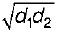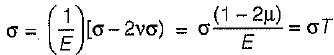Courses

# Test: Properties Of Metals, Simple Stress Strain And Elastic Constants - 3

## 20 Questions MCQ Test Topicwise Question Bank for GATE Civil Engineering | Test: Properties Of Metals, Simple Stress Strain And Elastic Constants - 3

Description
This mock test of Test: Properties Of Metals, Simple Stress Strain And Elastic Constants - 3 for Civil Engineering (CE) helps you for every Civil Engineering (CE) entrance exam. This contains 20 Multiple Choice Questions for Civil Engineering (CE) Test: Properties Of Metals, Simple Stress Strain And Elastic Constants - 3 (mcq) to study with solutions a complete question bank. The solved questions answers in this Test: Properties Of Metals, Simple Stress Strain And Elastic Constants - 3 quiz give you a good mix of easy questions and tough questions. Civil Engineering (CE) students definitely take this Test: Properties Of Metals, Simple Stress Strain And Elastic Constants - 3 exercise for a better result in the exam. You can find other Test: Properties Of Metals, Simple Stress Strain And Elastic Constants - 3 extra questions, long questions & short questions for Civil Engineering (CE) on EduRev as well by searching above.
QUESTION: 1

Solution:
QUESTION: 2

Solution:
QUESTION: 3

### If the cross-section of a member is subjected to a uniform shear stress of intensity ‘q’, then the strain energy stored per unit volume is equal to (G = modulus of rigidity)

Solution:
QUESTION: 4

In the case of an engineering material under unidirectional stress in the x-axis, the Poisson’s ratio is equal to (symbols have their usual meanings)

Solution:
QUESTION: 5

A 100 mm long and 50 mm diameter steel rod fits snugly between two rigid walls 100 mm apart at room temperature. Young's modulus of elasticity and coefficient of linear expansion of steel are 2 x 105 N/mm2 and 12 x 10-6/°C respectively. The stress developed in the rod due to a 100°C rise in temperature will be

Solution:

l = 100 mm, d = 50 mm,
E = 2 x 105 N/mm2, α = 12 x 10-6/°C
δl = αlΔT, ε = δl/l, σ = E = Eα
= 2 x 105 x 12 x 10-6 x 100 = 240 N/mm2

QUESTION: 6

During tensile testing of a specimen using a Universal Testing Machine, the parameters actually measured include

Solution:
QUESTION: 7

If the value of:Poisson’s ratio is zero, then it means that

Solution:
QUESTION: 8

The stretch in a steel rod of circular section, having a length ℓ subjected to a tensile load P and tapering uniformly from a diameter d1, at one end to a 'diameter d2 at the other end, is given by

Solution:
QUESTION: 9

If Poisson’s ratio for a material is 0.5, then the elastic modulus for the material is

Solution:

E = 2G(1 + μ) or E/G = 2 x 1.5 =3

QUESTION: 10

The Poisson’s ratio of a material which has Young’s modulus of 120 GPa, and shear modulus of 50 GPa, is

Solution:

G = E/2(1+μ)
or, 1 + μ = 120/(2 x 50) = 1.2
∴ μ = 0.2

QUESTION: 11

A rod of material E = 200 x 103 MPa and α = 10-3 mm/mm/°C is fixed at both the ends. It is uniformly heated such that the increase in temperature is 30°C. The stress developed in the rod is

Solution:

σ = E ∝ T = -200 x 103 x 10-3 x 30
= -6000N/mm2

QUESTION: 12

The deformation of a bar under its own weight as compared to that when subjected to a direct axial load equal to its own weight will be

Solution:
QUESTION: 13

The number of independent elastic constants required to express the stress-strain relationship for linearly elastic isotropic materia! is

Solution:

There are two independent elastic constants E and G for an isotropic linear elastic material.

QUESTION: 14

A tapering bar (diameters of end sections being d1 and d2) and a bar of uniform cross-section ‘d' have the same length and are subjected to the same axial pull. Both the bars will have the same extension if ‘ d ’ is equal to

Solution:

δteper = 4Pl(πd1/d2E)
δuniform = 4Pl/(pd2 E)
∴ d =QUESTION: 15

The number of elastic constants for a completely anisotropic elastic material which follows Hooke’s law is

Solution:
QUESTION: 16

For a given material, the modulus of rigidity is 100 GPa and Poisson’s ratio is 0.25. The value of modulus of elasticity in GPa is

Solution:

E = 2G (1 + μ) = 2 x 100(1 + 0.25)
= 250 GPa

QUESTION: 17

A cube having each side of length ‘a’ is constrained in all directions and is heated uniformly so that the temperature is raised to T°C. If α is the thermal coefficient of expansion of the cube material and E is the modulus of elasticity, the stress developed in the cube is

Solution:

For hydrostatic state stress = 0
σx = σy = σz = 0σ = EαT/1-2μ

QUESTION: 18

Toughness for mild steel under uniaxial tensile loading is given by the shaded portion of the stress-strain diagram as shown in

Solution:

Toughness is the total area under the stress- strain curve upto fracture.

QUESTION: 19

Which one of the following is correct in respect of Poisson’s ratio (v) limits for an isotropic elastic solid?

Solution:
QUESTION: 20

A bar of copper and steel form a composite system. They are heated to a temperature of 40°C. What type of stress is induced in the copper bar?

Solution:

Coefficient of expansion of copper is more than that of steei. Hence, copper will develop compressive stress.